Hardwood (Light)

Hardwoods are great because of there slow decomposition rate. Like all our mulches, they help retain moisture, reduce weeds, and also help protect your plants and trees from disease.

• Our lightest hardwood mulch
• Great for weed suppression
• Great for any flower bed or base of a new tree
• Effective in repelling insects and fungus
If you need help finding out your total cubic yardage use one of our calculators below!
Aerial Map Calculator
Area Totals
Current Area
0 ft2
Total Area
0 ft2
Total Measurement: 0 cubic yards
Total Price: \$0
Square Footage Calculator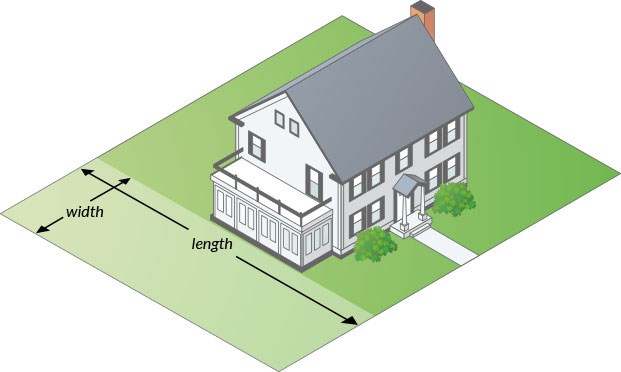How to measure your area for mulch

• Area 1
• Area 1
• Area 1
Total Measurement: 0 cubic yards
Total Price: \$0

How To Measure

Square or Rectangle

Measure the Length & Width and multiply.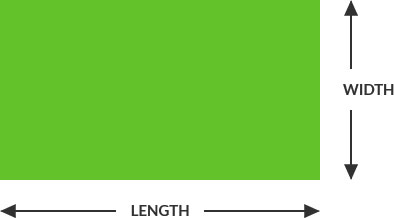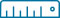Length x Width = Area
(12’ x 24’ = 288 ft2)

Right Triangle

Multiply the two sides that contact the right angle and divide by two.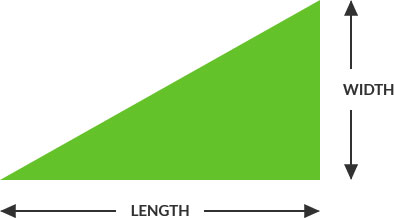Length x Width / 2 = Area
(12’ x 24’ = 288 ÷ 2 = 144 ft2)

Circular

Multiply the radius with the radius then multiply by 3.14. Radius is a straight line from the center of a circle to the outside of the circle.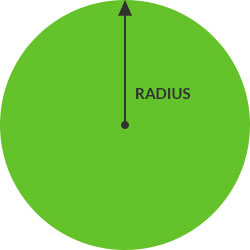Full Circle:(6’ x 6’ = 36 x 3.14 = 113 ft2)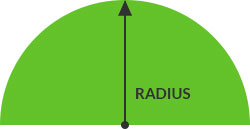Half Circle:Divide full circle radius by 2
(6’ x 6’ = 36 x 3.14 = 113 / 2 = 56.5 ft2)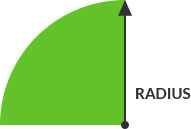Quarter Circle:Divide full circle radius by 4
(6’ x 6’ = 36 x 3.14 = 113 / 4 = 28.25 ft2)